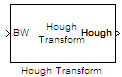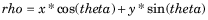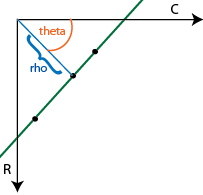# Hough Transform

Find lines in images

•Libraries:
Computer Vision Toolbox / Transforms

## Description

Use the Hough Transform block to find straight lines in an image. The block outputs the Hough space matrix and, optionally, the rho-axis and theta-axis vectors. Peak values in the matrix represent potential straight lines in the input image. You can use the output of the Hough Transform block to find straight lines in the image by using the Hough Lines block. Alternatively, you can use a custom algorithm to identify potential straight lines by locating peaks in the Hough space matrix.

## Ports

### Input

expand all

Binary image, specified as a matrix of `Boolean` values.

Data Types: `Boolean`

### Output

expand all

Parameter space matrix, returned as a numeric matrix. The values in the parameter space matrix are in the (rho, theta) space.

Data Types: `single` | `double` | `fixed point`

Theta values, returned as a numeric vector. Each element represents the rotation angle of the corresponding line, in radians.

#### Dependencies

To enable this port, you must select the Output theta and rho values parameter.

Data Types: `single` | `double` | `fixed point`

Rho values, returned as a numeric vector. Each element represents the distance of the corresponding line from the top-left point of the image.

#### Dependencies

To enable this port, you must select the Output theta and rho values parameter.

Data Types: `single` | `double` | `fixed point`

## Parameters

expand all

### Main

Specify the spacing of the Hough transform bins along the theta-axis. This value must be less than `pi/2`.

Specify the spacing of the Hough transform bins along the rho-axis.

Select this parameter to enable the Theta and Rho output ports. The block outputs the theta-axis and rho-axis vectors, respectively, at these ports.

Specify the data type of your output signal. If you select `double` or `single`, all the outputs are of the specified data type. If you select `Specify via Fixed-point tab`, you can specify the word length of each output in the Data Types tab.

### Data Types

For details on the fixed-point block parameters, see Specify Fixed-Point Attributes for Blocks (DSP System Toolbox).

Select this parameter to prevent the fixed-point tools from overriding the data types you specify in this block. For more information, see Lock the Output Data Type Setting (Fixed-Point Designer).

## Block Characteristics

 Data Types `Boolean` | `doublea` | `fixed point` | `integer` | `singlea` Multidimensional Signals `no` Variable-Size Signals `yes` a This data type is supported at the output port(s).

## Algorithms

The Hough Transform block implements the Standard Hough Transform (SHT). The SHT uses the parametric representation of a line:The block uses the upper-left corner pixel of the image as the origin, assigning it the xy-value of (0, 0).

The rho value indicates the perpendicular distance from the origin to the Hough line.

The theta value indicates the angle of inclination of the normal line from the x-axis. The range of theta is $-\frac{\pi }{2}\le \theta <+\frac{\pi }{2}$, with a step-size determined by the Theta resolution (radians) parameter. The SHT measures the angle of the line clockwise, with respect to the positive x-axis.

The Hough Transform block creates an accumulator matrix. The (rho, theta) pair represent the location of a cell in the accumulator matrix. Every valid (logical `true`) pixel of the input binary image represented by (row, col) produces a rho value for all theta values. The block quantizes the rho values to the nearest number in the rho vector. The rho vector depends on the size of the input image and the user-specified rho resolution. The block increments a counter (initially set to zero) in those accumulator array cells represented by the (rho, theta) pairs found for each pixel. This process validates that the point (row, col) is on the line defined by (rho, theta). The block repeats this process for each logical `true` pixel in the image. The Hough Transform block outputs the resulting accumulator matrix.

## Version History

Introduced before R2006a